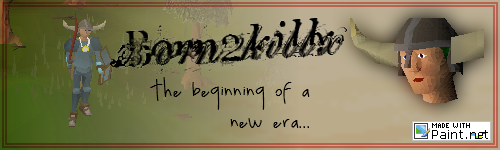# Image Transformation

## Recommended Posts

Here, I am not talking about the interface, but the math behind it.

If we want to start a free transform with smooth curves, we'll need to break out the calculus (unless there's another way?), but let's not go there yet.

Okay. Now we have here a 10x10 selection. There are 100 pixels here.Each one of these borders is describeable by a function (offsets not included...)And even a skewed selection (shown here in red), is defineable. (The grid is supposed to be skewed also, but if I could do that, why would I post this?)Now, as to rendering a product.

Four pixels here are contributing to one pixel.Let (line) 1 be defined by y=-2x. Let (line) 2 be defined by y=.5x. Let it be known that the pixel has dimensions 1 by 1 units. Say that line 2 starts at 0.6 here. (y=0.5x-0.6) And say that 1 starts at .6 also. (y=-2x+1.2). Breaking the quadrilaterals into triangles by drawing lines from p3 to the vertices defining the square pixel, we can calculate the area.What we are going to go to here is Heron's formula, no telling what kind of triangles one might find. So first, define the semiperimeter of a triangle. Let's go with the very top left influencing pixel's triangle to the left (image higlighting).Okay, so we need to know the perimeter. What defines the bounds of the triangle? The functions. Get your dusty distance formula out of your head. It'll come handy here in a second.

Okay. Where is p3?

Now, if we will be programming this, we need to find a way to do the algebra. Since all equations will be in mx+b form, here is how it will work:

mx+b=Mx+B

mx=Mx+B-b

(m-M)x=B-b

x = (B-b)/(m-M)

Now to find y, take your function, except now x is defined.

y=mx'+b

Now, where does the function y=0.5x-0.6 intersect x=0?

y=m0+b

y=b

Simple, however, when you are dealing with a pixel not on the first column, say x=312, you need this:

y=(m*312)+b

Simple still.

Okay, now we need to know the other point, the corner over there. With some logic, since our pixel is defined by x=1 and y=1, and we subract one to equal out human adhustment, we see that that point is {0, 0}.

Okay, remember when I said break out the distance formula? Time to kill computing time! Okay. x and y is the intersection of the two lines defining the pixel. x' and y' are where y=0.5x-0.6 intersects x=0. x'' and y'' are the top left corner.

a = sqrt(((x - x')^2)+((y-y')^2))

b = sqrt(((x' - x'')^2)+((y' - y''))

c = sqrt(((x''-x)^2)+((y''-y^2)))

Now for the semiperimeter:

s = (a+b+c)/2

And the area:

area = sqrt(s(s-a)(s-(s-c))

Okay, skip along a while. You've added the triangle values, and you find that the influencing pixels' areas in the future pixel are:

Top left: .22

Top right: .26

Bottom left: .27

Bottom right: .25

And let's say that the pixels colors are:

Top left: 255, 0, 0

Top right: 255, 72, 31

Bottom left: 255, 58, 96

Bottom right: 210, 32, 47

Here is a quick equation to find your pixel:

p.R = (tl.R*tl_Area)+(tr.R*tr_Area)+(bl.R*bl_Area)+(br.R*br_Area)

p.B = (tl.B*tl_Area)+(tr.B*tr_Area)+(bl.B*bl_Area)+(br.B*br_Area)

p.G = (tl.G*tl_Area)+(tr.G*tr_Area)+(bl.G*bl_Area)+(br.G*br_Area)

p.A = (tl.A*tl_Area)+(tr.A*tr_Area)+(bl.A*bl_Area)+(br.A*br_Area)

Apart from some logic problems, this is a page outlining the basics.

~~

##### Share on other sites

ok.... AAAAAAAAAAAAHHH! *runs away screaming*##### Share on other sites

This is more of an Overflow topicMoved

The Paint.NET Blog: https://blog.getpaint.net/

Donations are always appreciated! https://www.getpaint.net/donate.html

##### Share on other sites

My brain exploded.I built my first computer at age 11!

##### Share on other sites

My brain exploded.

Clean up on aisle 4 please.

The Paint.NET Blog: https://blog.getpaint.net/

Donations are always appreciated! https://www.getpaint.net/donate.html

##### Share on other sites

LMAO...

Sorry...that is just funny today for some odd reason (Must've been that thing I saw at Kroger this morning...)

Oh...P.S. I could also give you the math about a free transform (smooth)....but I don't think you want to see integral calculus, today, do you?

~~

## Join the conversation

You can post now and register later. If you have an account, sign in now to post with your account.×   Pasted as rich text.   Paste as plain text instead

Only 75 emoji are allowed.

×   Your previous content has been restored.   Clear editor

×   You cannot paste images directly. Upload or insert images from URL.

×
×